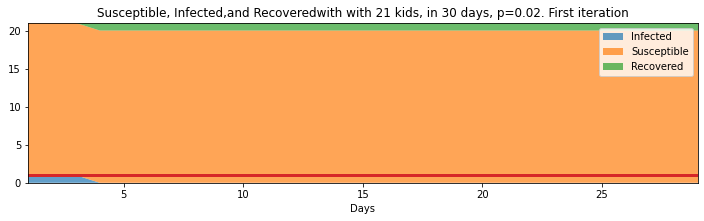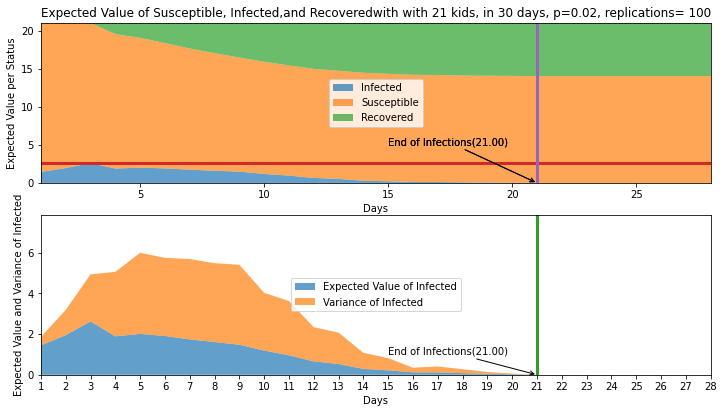This paper studies a pandemic flu spread case applying a “green” simulation method, using pseudo-random numbers as presented in the paper by Wilson S., Alabdulkarim A. and Goldsman D. W, “Green Simulation of Pandemic Disease Propagation” in a simulation environment build in Python. The scenario is an elementary school with twenty-one kids and the impact of infection when one infected kid enters the system. The findings and answers to the questions are presented at the end of the paper. The paper can be read here.

## Modeling

This section creates the replications. The input here are: The number of kids, the number of days and the probability of infection

```replications=100
kids=21
days=30
p=0.02
#initializes the data frame of replications
summary_df_replications = pd.DataFrame(data= {'day':[], 'infected':[], 'susceptible':[],'recovered':[],
'probability':[], 'count_prn':[] })
#initializes the list of last recovered which I will be saving in a data frame for every iteration
summary_last_recovered=summary_df_replications

mean_per_day =pd.DataFrame(data= {'day':[], 'infected':[], 'susceptible':[],'recovered':[],
'probability':[], 'count_prn':[] })

#runs every iteration
for i in range (0, replications):
metrics_result, info_last_recovered = pandemic (kids, days+1, p )
#n number of students, k number of days and p probability
#in this case 21 students with a lenght of 365 days and probability of 0.02

#creates a single data frame for all replications
summary_df_replications=pd.concat([summary_df_replications, pd.DataFrame(data=metrics_result)])
#creates a single data frame for all replications last recovered
summary_last_recovered=pd.concat([summary_last_recovered, pd.DataFrame(data=info_last_recovered)])

```
```def getDayNoInfection(df, infected='infected'):
counter = 0
index = 0
for index,d in df.iterrows():
if d[infected] == 0:
counter = counter + 1
if counter == 0:
index = d['day']
if counter >= 3:
break
return index-1
def getDaysOfNoInfectionsMean(df):
days_change = []
for i in range(replications):
day = getDayNoInfection(df[i*60: i*60 + 60])
days_change.append(day)
out= sum(days_change)/len(days_change)
#print(out)
return (out, days_change)

last_infected= getDaysOfNoInfectionsMean(summary_df_replications)
#print(counter)
```

## Results

```#show the table of summary_df_replications
#print(summary_df_replications[0:days])
print ("Table of Replications")
display(summary_df_replications)

#testing to see the tail of the entire dataframe
#print (summary_df_replications.tail())
#test to see data from the daya of last recovered
print ("Last Day of The Iteration per Replication")
display(summary_last_recovered)
```
```Table of Replications
```
day infected susceptible recovered probability count_prn
0 1.0 1.0 20.0 0.0 0.02 20.0
1 2.0 1.0 20.0 0.0 0.02 40.0
2 3.0 1.0 20.0 0.0 0.02 60.0
3 4.0 0.0 20.0 1.0 0.00 80.0
4 5.0 0.0 20.0 1.0 0.00 100.0
... ... ... ... ... ... ...
25 26.0 0.0 14.0 7.0 0.00 393.0
26 27.0 0.0 14.0 7.0 0.00 407.0
27 28.0 0.0 14.0 7.0 0.00 421.0
28 29.0 0.0 14.0 7.0 0.00 435.0
29 30.0 0.0 14.0 7.0 0.00 449.0

3000 rows × 6 columns

```Last Day of The Iteration per Replication
```
day infected susceptible recovered probability count_prn
0 30.0 0.0 20.0 1.0 0.0 600.0
0 30.0 0.0 17.0 4.0 0.0 520.0
0 30.0 0.0 6.0 15.0 0.0 318.0
0 30.0 0.0 7.0 14.0 0.0 329.0
0 30.0 0.0 13.0 8.0 0.0 446.0
... ... ... ... ... ... ...
0 30.0 0.0 20.0 1.0 0.0 600.0
0 30.0 0.0 16.0 5.0 0.0 492.0
0 30.0 0.0 15.0 6.0 0.0 472.0
0 30.0 0.0 6.0 15.0 0.0 303.0
0 30.0 0.0 14.0 7.0 0.0 449.0

100 rows × 6 columns

```summary_last_recovered.mean()
```
```day             30.00
infected         0.00
susceptible     14.03
recovered        6.97
probability      0.00
count_prn      457.01
dtype: float64```

```import matplotlib.pyplot as plt
import seaborn as sns
fig = plt.figure(figsize=(12, 10))

########## Plotting the first iteration #################

pal = sns.color_palette("tab10")
max_infected=max(summary_df_replications["infected"][0:days])
#top plot
ax.stackplot(summary_df_replications["day"][0:days],
summary_df_replications["infected"][0:days],
summary_df_replications["susceptible"][0:days],
summary_df_replications["recovered"][0:days],
colors=pal, alpha=0.7)
ax.set_title(f'Susceptible, Infected,and Recoveredwith with {kids} kids, in {days} days, p={p}. First iteration')
ax.set_xlabel('Days')
ax.legend([
'Infected',
'Susceptible',
'Recovered'],
loc='best')
ax.set_xlim(1, days-1)
ax.set_ylim(0, kids)

#this plots a line with the max infected
ax.annotate("Max infected(%.2f)"%(max_infected),
(0, max_infected),(0+3, max_infected+3),
#(max_infected, max_infected),
arrowprops=dict(arrowstyle='->'))
ax.plot(np.array([0, days-1]), [max_infected, max_infected], lw=3, label='Max Infected')
```

`[<matplotlib.lines.Line2D at 0x16b297baf88>]````summary_df_replications_Table= summary_df_replications.rename(columns={"day": "Day","infected":"Infected", "susceptible":"Susceptible",
"recovered":"Removed","probability": "Probability",
"count_prn": "Cumulative PRNs" } )
#summary_df_replications_Table.iloc[0:int(last_infected)]
#type summary_df_replications_Table = summary_df_replications_Table.astype({"Day": int, "Infected": int})
```

```##################### Calculating the Means for each day ####################
#calculates the mean per replications
#not correcto start
mean_replications = pd.DataFrame(summary_last_recovered.mean(axis=0)).T
mean_replications
# not correcto end

#mean of first iteration
#print(summary_df_replications["infected"][0:days].mean())

#mean of the day 1
#print(f'Mean of day 1 :{summary_df_replications["infected"].mean()}')

#mean of all days 1
mean_of_days_infected=[]
mean_of_days_susceptible=[]
mean_of_days_removed=[]

#calculating the variance per day
var_of_days_infected=[]
var_of_days_susceptible=[]
var_of_days_removed=[]

#creating a table only for the infected values
#if the mean is taking for all of the days including the 0 the mean will also include zero values

#set the range of the days

x=range(1,days)

for i in range(0,days-1):
mean_of_days_infected.append(summary_df_replications["infected"][i].mean())
mean_of_days_susceptible.append(summary_df_replications["susceptible"][i].mean())
mean_of_days_removed.append(summary_df_replications["recovered"][i].mean())
#for the variance
var_of_days_infected.append(summary_df_replications["infected"][i].var())
var_of_days_susceptible.append(summary_df_replications["susceptible"][i].var())
var_of_days_removed.append(summary_df_replications["recovered"][i].var())

#print(summary_df_replications["infected"][i].mean())

#print(f'mean of each day {mean_of_days_infected}')
dataframe_means= pd.DataFrame(data={'Day':x,
'mean_per_day_infected':mean_of_days_infected,
'mean_of_days_susceptible':mean_of_days_susceptible,
'mean_of_days_removed':mean_of_days_removed})
#creates a dataframeof the variances
dataframe_variance=pd.DataFrame(data={'Day':x,
'Variance per day Infected':var_of_days_infected,
'Variance per day Susceptible':var_of_days_susceptible,
'Variance per day Recovered':var_of_days_removed} )
```

```last_infected_mean= getDayNoInfection(dataframe_means,'mean_per_day_infected')
print(f'Day that no more kids gets infected {last_infected_mean}th day')
dataframe_means
print()
mean_days, array_days= getDaysOfNoInfectionsMean(summary_df_replications)
End_total_infection = summary_df_replications[summary_df_replications["infected"]<1]
#End_total_infection[]
#getDaysOfNoInfectionsMean(summary_df_replications)
```
```Day that no more kids gets infected 21th day

```

```#this plots the info in a stack
fig = plt.figure(figsize=(12, 10))

plt.xticks(np.arange(min(x), max(x)+1, 1.0))

max_infected_means=max(dataframe_means["mean_per_day_infected"][0:days])
print(max_infected_means)
ax.stackplot(dataframe_means["Day"][0:days],
dataframe_means["mean_per_day_infected"][0:days],
dataframe_means["mean_of_days_susceptible"][0:days],
dataframe_means["mean_of_days_removed"][0:days],
colors=pal, alpha=0.7)

ax.set_title(f'Expected Value of Susceptible, Infected,and Recoveredwith with {kids} kids, in {days} days, p={p}, replications= {replications}')
ax.set_xlabel('Days')
ax.set_ylabel('Expected Value per Status')
ax.legend([
'Infected',
'Susceptible',
'Recovered'],
loc='center')
ax.set_xlim(1, days-2)

ax.set_ylim(0, kids)

#this plots a line with the max infected
ax.annotate("Max infected(%.2f)"%(max_infected_means),
(0, max_infected_means),(0+3, max_infected_means+3),
#(max_infected, max_infected),
arrowprops=dict(arrowstyle='->'))
ax.plot(np.array([0, days-1]), [max_infected_means, max_infected_means], lw=3, label='Max Infected Means')

#this plots a line with the last day infected
ax.annotate("End of Infections(%.2f)"%(last_infected_mean),
(last_infected_mean, 0),(last_infected_mean-6, 0+5),
#(max_infected, max_infected),
arrowprops=dict(arrowstyle='->'))
ax.annotate("End of Infections(%.2f)"%(last_infected_mean),
(last_infected_mean, 0),(last_infected_mean-6, 0+5),
#(max_infected, max_infected),
arrowprops=dict(arrowstyle='->'))
ax.plot(np.array([last_infected_mean,last_infected_mean]), [0, kids], lw=3, label='Last Infected Means')

#############################################################################################
#This plot is for the infected on the sencond part
ax.set_xlim(1, days-2)
ax.set_ylim(0, max_infected_means*3)

ax.stackplot(x,
dataframe_means["mean_per_day_infected"][0:days-1],
dataframe_variance["Variance per day Infected"][0:days-1],
colors=pal, alpha=0.7)
ax.legend([
'Expected Value of Infected',
'Variance of Infected'],
loc='center')
#this plots a vertical line of end of infection in plot 2
ax.annotate("End of Infections(%.2f)"%(last_infected_mean),
(last_infected_mean, 0),(last_infected_mean-6, 0+1),
#(max_infected, max_infected),
arrowprops=dict(arrowstyle='->'))
ax.plot(np.array([last_infected_mean,last_infected_mean]), [0, kids], lw=3, label='Last Infected Means')

#sets x title
ax.set_xlabel('Days')
ax.set_ylabel('Expected Value and Variance of Infected')
```

```2.62
```
`Text(0, 0.5, 'Expected Value and Variance of Infected')`